# 4.3   Stacks and Queues

In this section, we introduce two closely-related data types for manipulating arbitrarily large collections of objects: the stack and the queue. Stacks and queues are special cases of the idea of a collection. Each is characterized by four operations: create the collection, insert an item, remove an item, and test whether the collection is empty.

## Stacks.

A stack is a collection that is based on the last-in-first-out (LIFO) policy. By tradition, we name the stack insert method push() and the stack remove operation pop(). We also include a method to test whether the stack is empty, as indicated in the following API: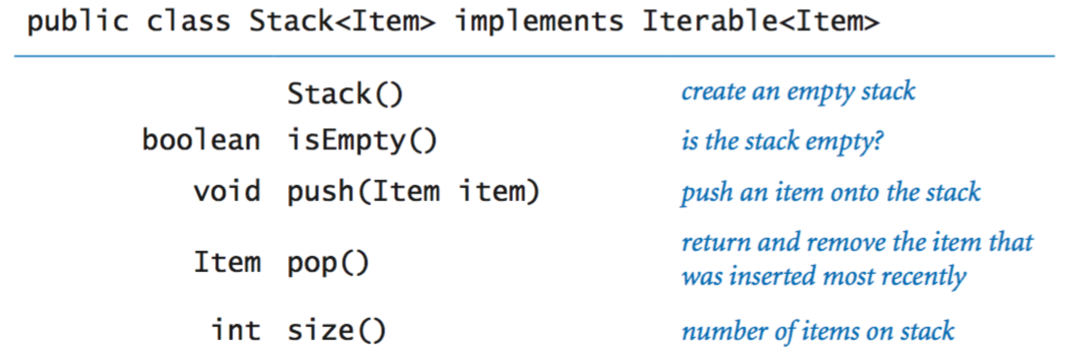## Array implementations of stacks.

Representing stacks with arrays is a natural idea. In particular, we maintain an instance variable n that stores the number of items in the stack and an array items[] that stores the n items, with the most recently inserted item in items[n-1] and the least recently inserted item in items. This policy allows us to add and remove items at the end without moving any of the other items in the stack.

• Fixed-length array implementation of a stack of strings. ArrayStackOfStrings.java implements this approach for a stack of strings whose maximum capacity is specified by the argument to the constructor. To remove an item, we decrement n and then return a[n]; to insert a new item, we set a[n] equal to the new item and then increment n.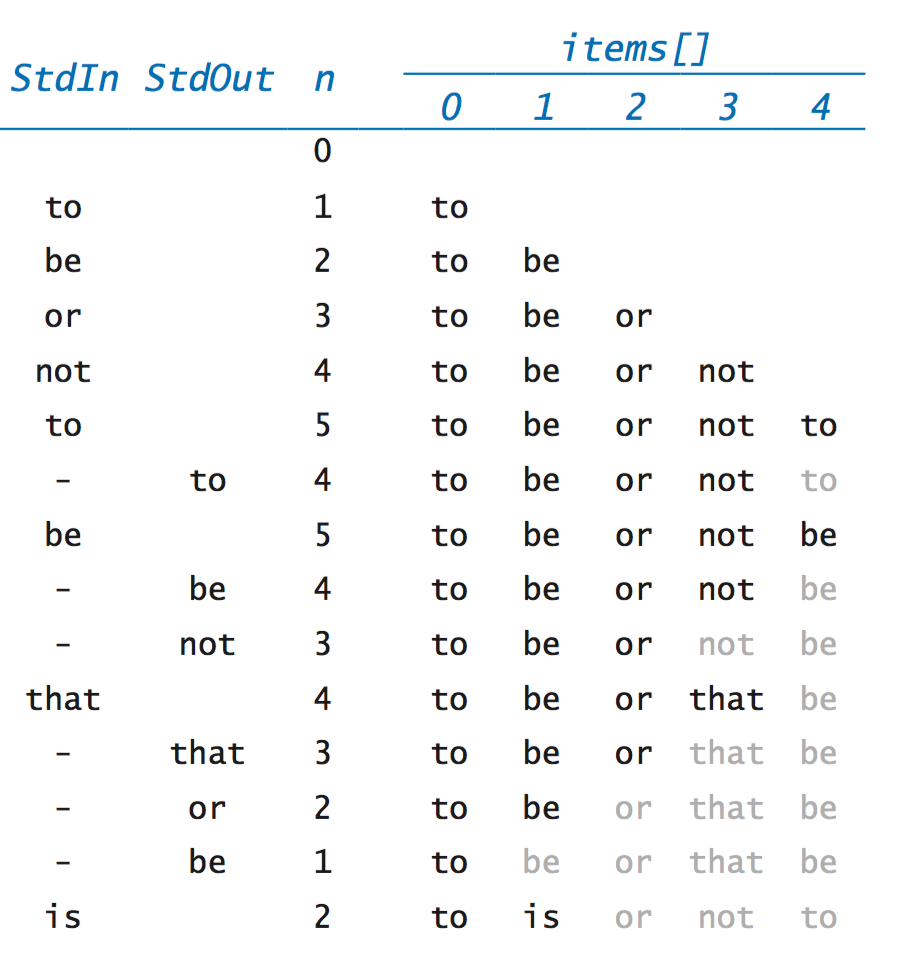• Resizing array implementation of a stack of strings. ResizingArrayStackOfStrings.java is a version of ArrayStackOfStrings.java that dynamically adjusts the length of the array items[] so that it is sufficiently large to hold all of the items and but not so large as to waste an excessive amount of space. First, in push(), we check whether there is room for the new item; if not, we create a new array of double the length of the old array and copy the items from the old array to the new array. Similarly, in pop(), we check whether the array is too large, and we halve its length if that is the case.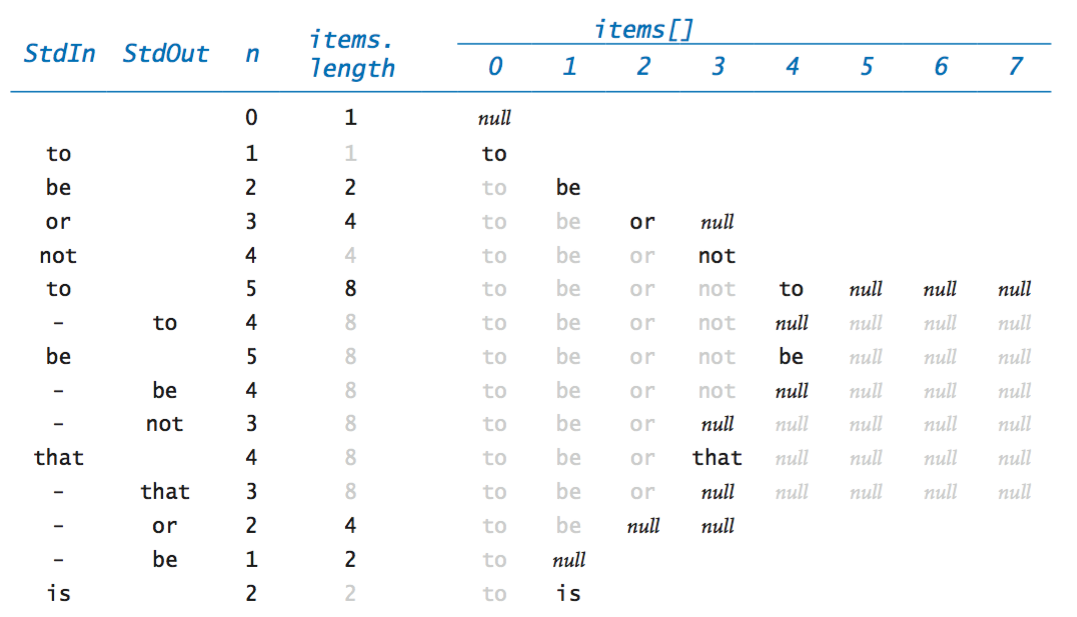This doubling-and-halving strategy guarantees that that the stack never overflows and never becomes less than one-quarter full.

• Resizing array implementation of a generic stack. ResizingArrayStack.java implements a generic stack using a resizing array. For technical reasons, a cast is needed when allocating the array of generics.

A singly linked list comprises a sequence of nodes, with each node containing a reference (or link) to its successor. By convention, the link in the last node is null, to indicate that it terminates the list. With object-oriented programming, implementing linked lists is not difficult. We define a class for the node abstraction that is recursive in nature:
 class Node { String item; Node next; }A Node object has two instance variables: a String and a Node. The String is a placeholder in this example for any data that we might want to structure with a linked list (we can use any set of instance variables); the instance variable of type Node characterizes the linked nature of the data structure.
• Linking together a linked list. For example, to build a linked list that contains the items "to", "be", and "or", we create a Node for each item: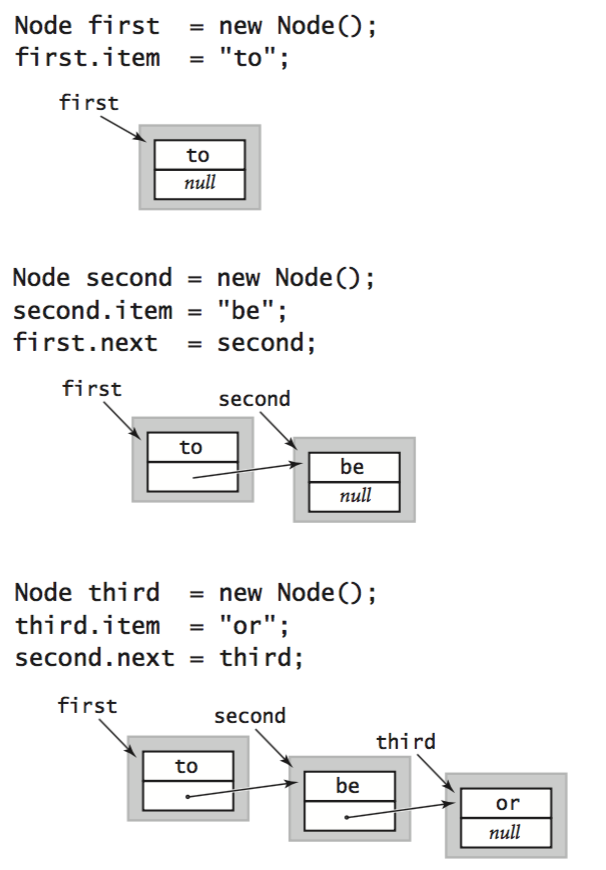• Insert. Suppose that you want to insert a new node into a linked list. The easiest place to do so is at the beginning of the list. For example, to insert the string not at the beginning of a given linked list whose first node is first, we save first in a temporary variable oldFirst, assign to first a new Node, and assign its item field to not and its next field to oldFirst.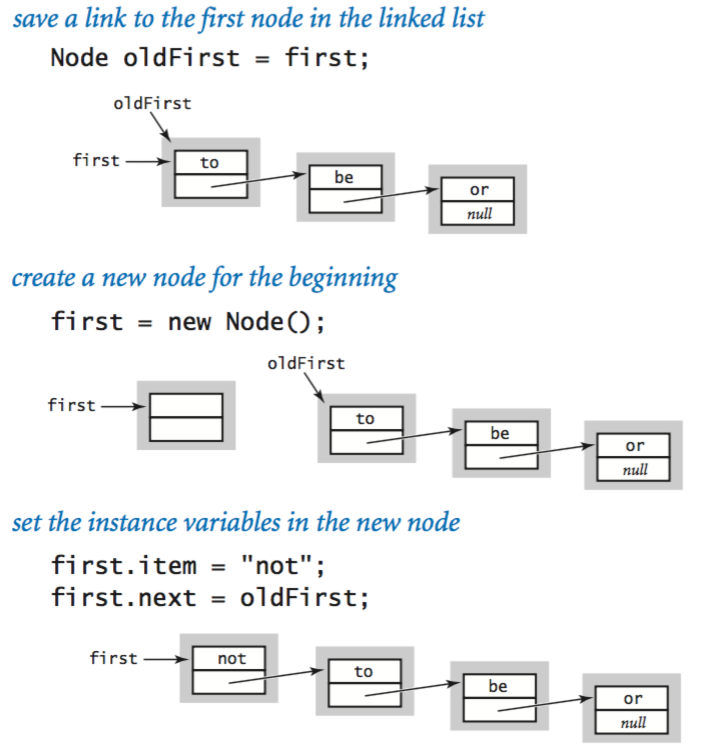• Remove. Suppose that you want to remove the first node from a list. This operation is even easier: simply assign to first the value first.next.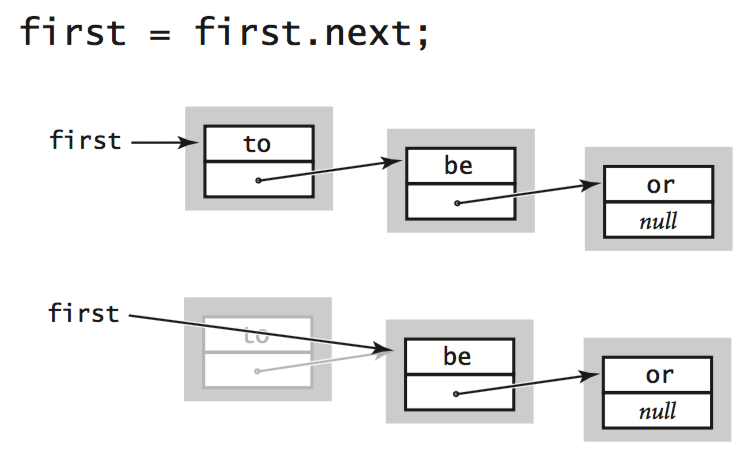• Traversal. To examine every item in a linked list, we initialize a loop index variable x that references the the first Node of the linked list. Then, we find the value of the item associated with x by accessing x.item, and then update x to refer to the next Node in the linked list, assigning to it the value of x.next and repeating this process until x is null (which indicates that we have reached the end of the linked list). This process is known as traversing the list, and is succinctly expressed in this code fragment:
 for (Node x = first; x != null; x = x.next) StdOut.println(x.item);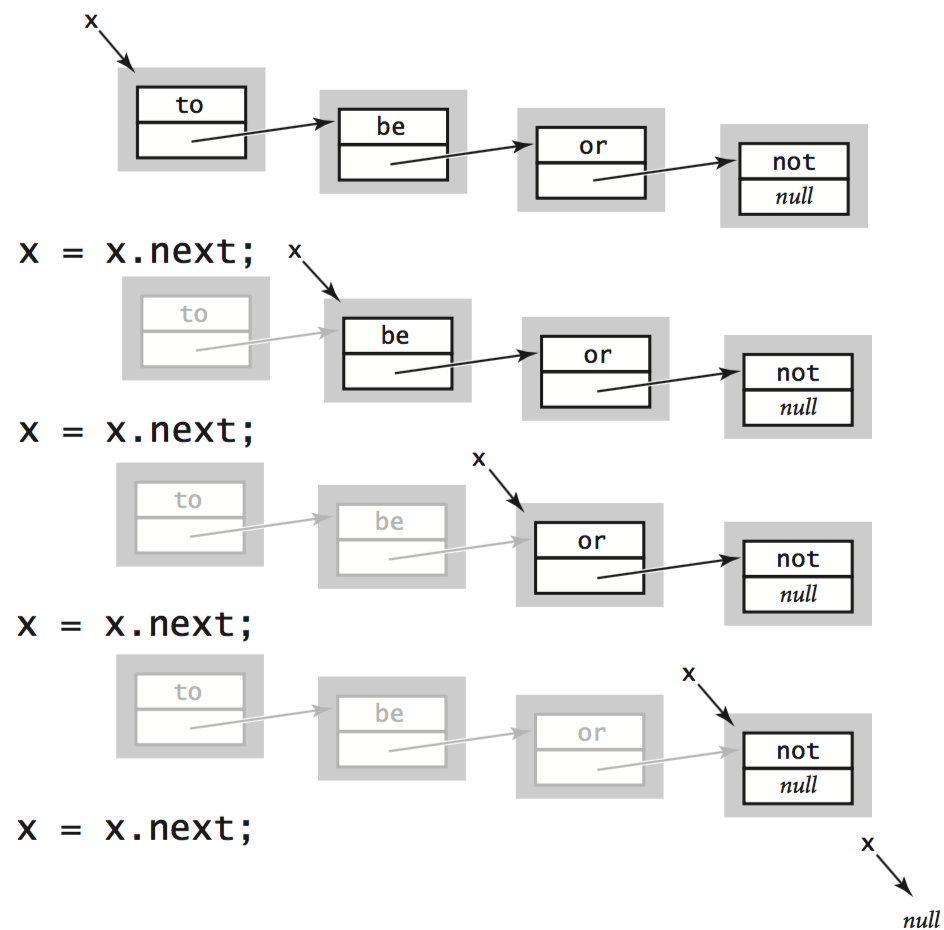## Implementing stacks with linked lists.

Representing stacks with linked lists is a natural idea. In particular, we maintain an instance variable first that stores a reference to the most recently inserted item. This policy allows us to add and remove items at the beginning of the linked list without accessing the links of any other items in the linked list.
• Linked-list implementation of a stack of strings. LinkedStackOfStrings.java uses a linked list to implement a stack of strings. The implementation is based on a nested class Node like the one we have been using. Java allows us to define and use other classes within class implementations in this natural way. We designate the nested class as private because clients do not need to know any of the details of the linked lists.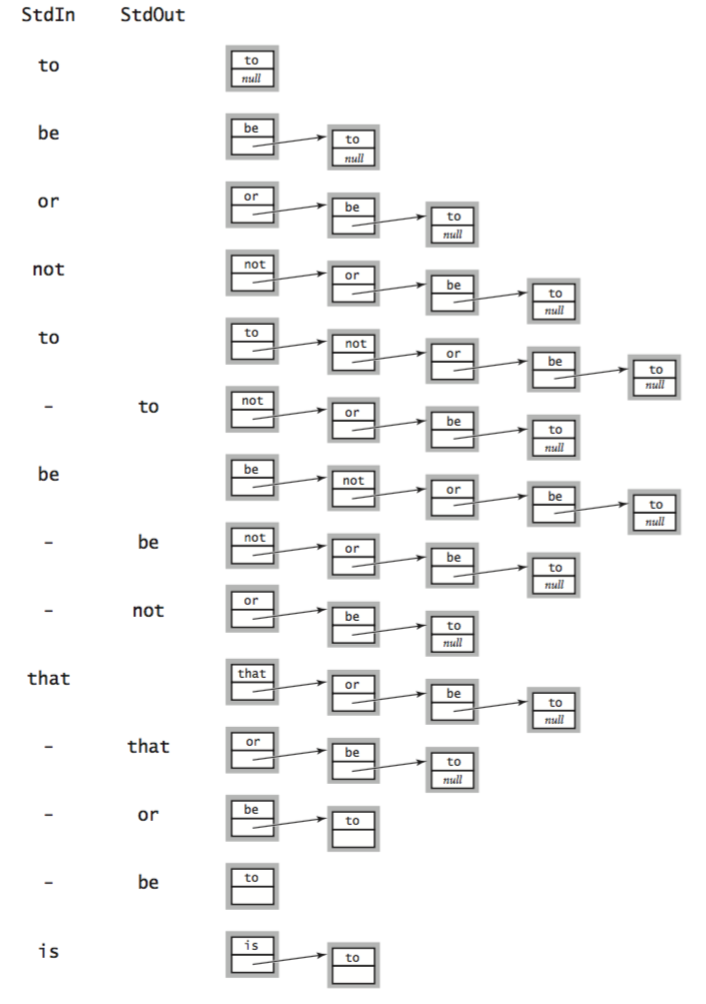• Linked-list implementation of a generic stack. Stack.java implements a generic stack using a singly linked list.

## Queue.

A queue supports the insert and remove operations using a first-in first-out (FIFO) discipline. By convention, we name the queue insert operation enqueue and the remove operation dequeue, as indicated in the following API: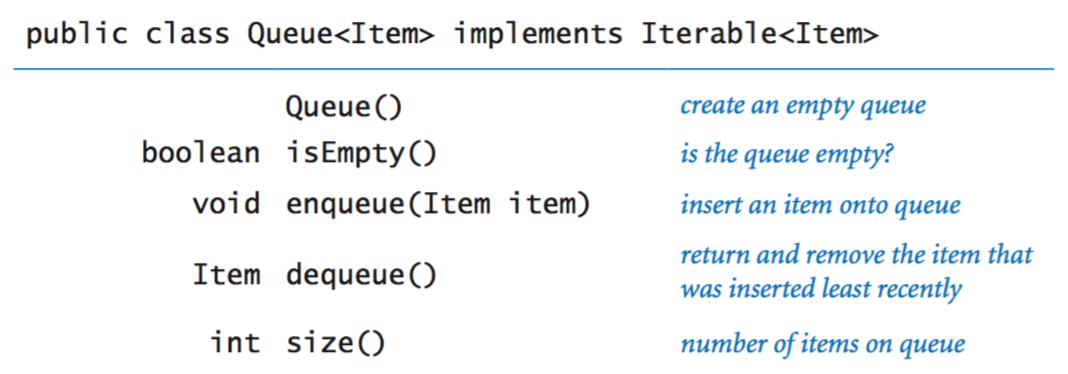• Linked-list implementation of a queue. Queue.java implements a FIFO queue of strings using a linked list. Like Stack, we maintain a reference first to the least-recently added Node on the queue. For efficiency, we also maintain a reference last to the most-recently added Node on the queue.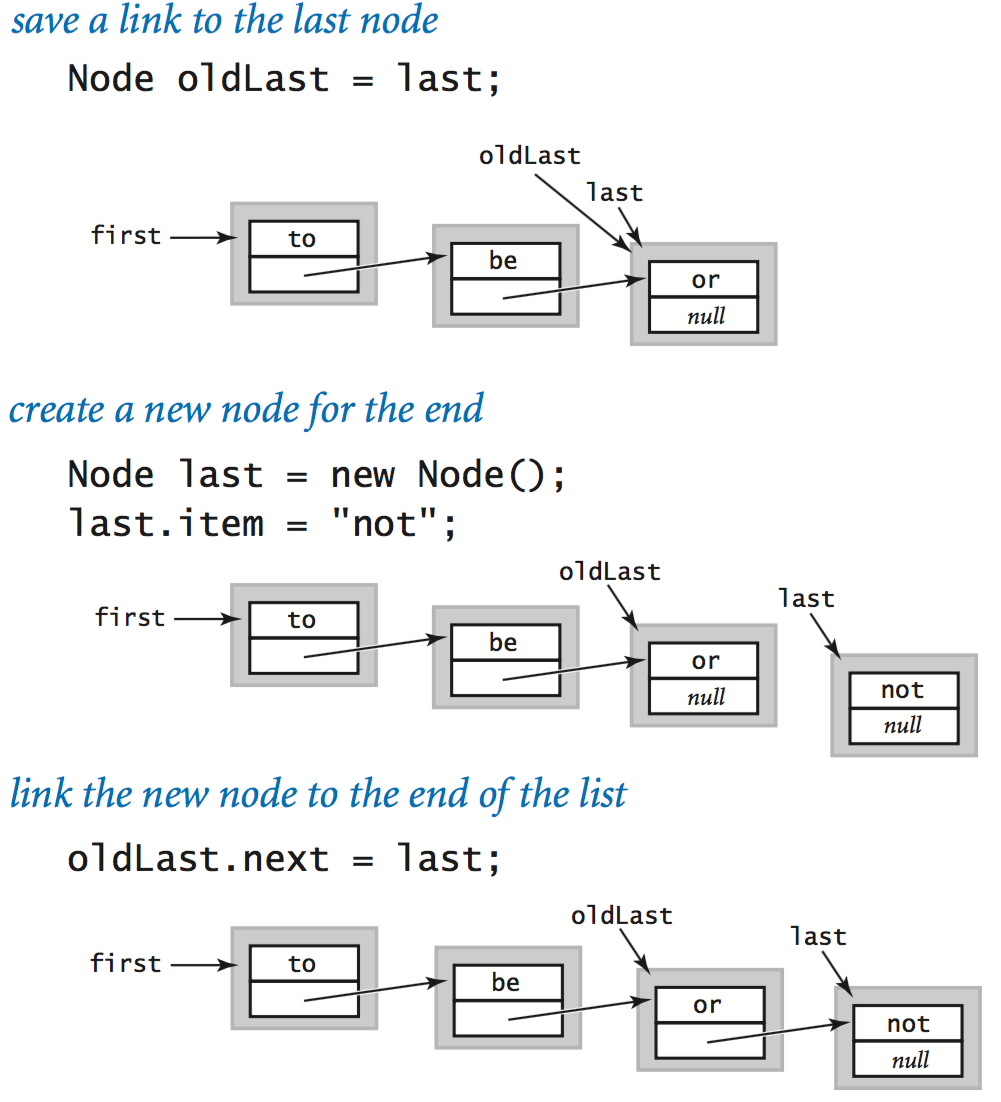• Resizing array implementation of a queue. ResizingArrayQueue.java implements a queue using a resizing array. It is similar to ResizingArrayStack.java, but trickier since we need to add and remove items from opposite ends of the array.### Generics.

We have developed stack implementations that allows us to build a stack of one particular type, such as String. A specific mechanism in Java known as generic types enables us to build collections of objects of a type to be specified by client code.
• Implementing a generic collection. To implement a generic collection, we specify a type parameter, such as Item, in angle brackets and use that type parameter in our implementation instead of a specific type. For example, Stack.java is generic version of LinkedStackOfStrings.java
• Using a generic collection. To use a generic collection, the client must specify the type argument when the stack is created:
 Stack stack = new Stack(); 

### Autoboxing.

We have designed our stacks to be generic, so that they objects of any type. The Java language features known as autoboxing and unboxing enable us to reuse generic code with primitive types as well. Java supplies built-in object types known as wrapper types, one for each of the primitive types: Boolean, Integer, Double, Character, and so forth. Java automatically converts between these reference types and the corresponding primitive types so that we can write code like the following:
 Stack stack = new Stack(); stack.push(17); // autoboxing (int -> Integer) int a = stack.pop(); // unboxing (Integer -> int) 

## Iteration.

Sometimes the client needs to access all of the items of a collection, one at a time, without deleting them. To maintain encapsulation, we do not want to reveal the internal representation of the queue (array or linked list) to the client. To accommodate this design pattern, Java provides the foreach statement. You should interpret the following for statement in the following code fragment as for each string s in the collection, print s.

 Stack collection = new Stack(); ... for (String s : stack) StdOut.println(s); 

Implementing a collection that supports iteration in this way requires implementing Java's java.util.Iterator and java.util.Iterable interfaces. See the textbook for details.

## Stack and queue applications.

Stacks and queues have numerous useful applications.

• Arithmetic expression evaluation. An important application of stacks is in parsing. For example, a compiler must parse arithmetic expressions written using infix notation. For example the following infix expression evaluates to 212.
 ( 2 + ( ( 3 + 4 ) * ( 5 * 6 ) ) ) 
Evaluate.java evaluates a fully parenthesized arithmetic expression.

• Function-call abstraction. Most programs use stacks implicitly because they support a natural way to implement function calls, as follows: at any point during the execution of a function, define its state to be the values of all of its variables and a pointer to the next instruction to be executed. The natural way to implement the function-call abstraction is to use a stack. To call a function, push the state on a stack. To return from a function call, pop the state from the stack to restore all variables to their values before the function call and resume execution at the next instruction to be executed.

• M/M/1 queue. One of the most important queueing models is known as an M/M/1 queue, which has been shown to accurately model many real-world situations. It is characterized by three properties:
• There is one server—a FIFO queue.
• Interarrival times to the queue obey an exponential distribution with rate λ per minute.
• Service times from a nonempty queue obey an exponential distribution with rate μ per minute.

MM1Queue.java simulates an M/M/1 queue and plots a histogram of waiting times to standard drawing.

• Load balancing. LoadBalance.java simulate the process of assigning n items to a set of m servers. For each item, it chooses a sample of s servers and assigns the item to the server that has the fewest current items.

#### Exercises

1. Add a method isFull() to ArrayStackOfStrings.java.
2. Write a filter Reverse.java that reads strings one at a time from standard input and prints them to standard output in reverse order.
3. Write a stack client Parentheses.java that reads a string of parentheses, square brackets, and curly braces from standard input and uses a stack to determine whether they are properly balanced. For example, your program should print true for [()]{}{[()()]()} and false for [(]).
4. What does the following code fragment print when n is 50? Give a high-level description of what the code fragment does when presented with a positive integer n.
 Stack stack = new Stack(); while (n > 0) { stack.push(n % 2); n /= 2; } while (!stack.isEmpty()) StdOut.print(stack.pop()); StdOut.println(); 

Solution: prints the binary representation of n (110010 when n is 50).

5. What does the following code fragment do to the queue queue?
 Stack stack = new Stack(); while (!queue.isEmpty()) stack.push(queue.dequeue()); while (!stack.isEmpty()) queue.enqueue(stack.pop()); 

Solution: reverses the order of the strings in the queue.

6. Add a method peek() to Stack.java that returns the most recently inserted element on the stack (without removing it).
7. Add a method size() to both Queue.java and Stack.java that returns the number of items in the collection.
8. Write a filter InfixToPostfix.java that converts an arithmetic expression from infix to postfix.
9. Write a program EvaluatePostfix.java that takes a postfix expression from standard input, evaluates it, and prints the value. (Piping the output of your program from the previous exercise to this program gives equivalent behavior to Evaluate.java.)
10. Develop a data type ResizingArrayQueueOfStrings.java that implements a queue wit ha fixed-length array in such a way that all operations take constant time.
11. Modify MM1Queue.java to make a program MD1Queue.java that simulates a queue for which the service times are fixed (deterministic) at rate μ. Verify Little's law for this model.
12. Develop a class StackOfInts.java that uses a linked-list representation (but no generics) to implement a stack of integers. Write a client that compares the performance of your implementation withStack<Integer> to determine the performance penalty from autoboxing and unboxing on your system.

1. Suppose x is a linked-list node. What is the effect of the following code fragment?
 x.next = x.next.next; 

Solution: Deletes from the list the node immediately following x.

2. Write a method delete() that takes the first node in a linked list and an int argument k and deletes the kth node in the linked list, if it exists.

Solution:

 // we assume that first is a reference to the first Node in the list public void delete(int k) { if (k <= 0) throw new RuntimeException("Invalid value of k"); // degenerate case - empty linked list if (first == null) return; // special case - removing the first node if (k == 1) { first = first.next; return; } // general case, make temp point to the (k-1)st node Node temp = first; for (int i = 2; i < k; i++) { temp = temp.next; if (temp == null) return; // list has < k nodes } if (temp.next == null) return; // list has < k nodes // change temp.next to skip kth node temp.next = temp.next.next; } 
3. Suppose that x is a linked-list node. What is the effect of the following code fragment?
 t.next = x.next; x.next = t; 

Solution: Inserts node t immediately after node x.

4. Why does the following code fragment not have the same effect as in the previous question?
 x.next = t; t.next = x.next; 

Solution: When it comes time to update t.next, x.next is no longer the original node following x, but is instead t itself!

#### Creative Exercises

1. Josephus problem. In the Josephus problem from antiquity, n people are in dire straits and agree to the following strategy to reduce the population. They arrange themselves in a circle (at positions numbered from 0 to n−1) and proceed around the circle, eliminating every mth person until only one person is left. Legend has it that Josephus figured out where to sit to avoid being eliminated. Write a Queue client Josephus.java that takes two integer command-line arguments m and n and prints the order in which people are eliminated (and thus would show Josephus where to sit in the circle).
2. Topological sort. You have to sequence the order of n jobs that are numbered 0 to n-1 on a server. Some of the jobs must complete before others can begin. Write a program TopologicalSorter.java that takes a command-line argument n and a sequence on standard input of ordered pairs of jobs (i, j), and then prints a sequence of integers such that for each pair (i, j) in the input, job i appears before job j. First, from the input, build, for each job (1) a queue of jobs that must follow it and (2) its indegree (the number of jobs that must come before it). Then, build a queue of all nodes whose indegree is 0 and repeatedly delete any job with a 0 indegree, maintaining all the data This process has many applications. For example, you can use it to model course prerequisites for your major so that you can find a sequence of courses to take so that you can graduate.
3. Copy constructor for a stack. Create a new constructor for the linked -list implementation of Stack.java so that Stack<String> t = new Stack<String>(s) makes t reference a new and independent copy of the stack s. You should be able to push and pop from either s or t without influencing the other.

Recursive solution: create a copy constructor for a Node and use this to create the new stack.

 public Node(Node x) { item = x.item; if (x.next != null) next = new Node(x.next); } public Stack(Stack s) { first = new Node(s.first); } 

Non-recursive solution (untested):

 public Node(Node x, Node next) { this.x = x; this.next = next; } public Stack(Stack s) { if (s.first != null) { first = new Node(s.first.value, s.first.next) { for (Node x = first; x.next != null; x = x.next) x.next = new Node(x.next.value, x.next.next); } } 
4. Quote. Develop a data type Quote.java that implements the following API: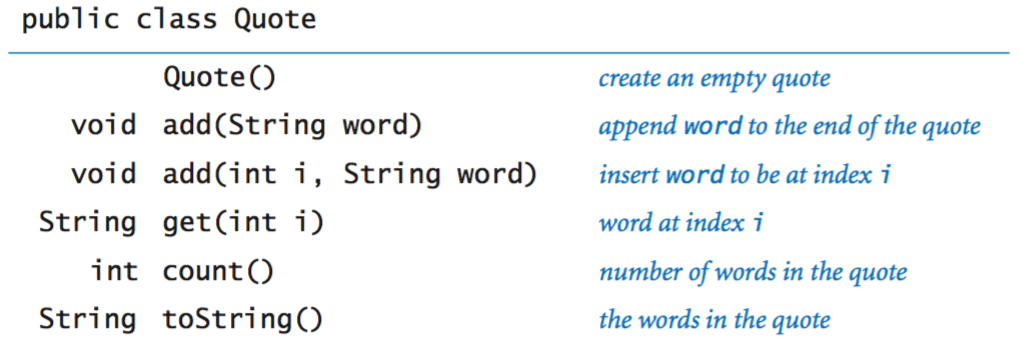To do so, define a nested class Card that holds one word of the quotation and a link to the next word in the quotation:
 private class Card { private String word; private Card next; public Card(String word) { this.word = word; this.next = null; } } 
5. Circular quote. Repeated the previous exercise, but use a circular linked list. In a circular linked list, each node points to its successor, and the last node in the list points to the first node (instead of null, as in a standard null-terminated linked list).

Solution: CircularQuote.java

6. Reverse a linked list (iteratively). Write a nonrecursive function that takes the first Node in a linked list as an argument, and reverses the list, returning the first Node in the result.

Solution: To accomplish this, we maintain references to three consecutive nodes in the linked list, reverse, first, and second. At each iteration we extract the node first from the original linked list and insert it at the beginning of the reversed list. We maintain the invariant that first is the first node of what's left of the original list, second is the second node of what's left of the original list, and reverse is the first node of the resulting reversed list.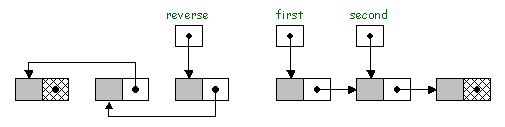public static Node reverse(Node list) { if (first == null || first.next == null) return first; Node first = list; Node reverse = null; while (first != null) { Node second = first.next; first.next = reverse; reverse = first; first = second; } return reverse; } 
7. Reverse a linked list (recursively). Write a recursive function that takes the first Node in a linked list as an argument and reverses the list, returning the first Node in the result.

Solution: Assuming the linked list has n elements, we recursively reverse the last n-1 elements, then append the first element to the end.

 public Node reverse(Node first) { if (first == null || first.next == null) return first; Node second = first.next; Node rest = reverse(second); second.next = first; first.next = null; return rest; } 
8. Listing files. A folder is a list of files an folders. Write a program Directory.java that takes the name of a folder as a command line argument and prints all of the files contained in that folder, with the contents of each folder recursively listed (indented) under that folder's name.

#### Web Exercises

1. Write a recursive function that takes as input a queue, and rearranges it so that it is in reverse order. Hint: dequeue() the first element, recursively reverse the queue, and the enqueue the first element.
2. Add a method Item[] multiPop(int k) to Stack that pops k elements from the stack and returns them as an array of objects.
3. Add a method Item[] toArray() to Queue that returns all N elements on the queue as an array of length N.
4. What does the following code fragment do?
 IntQueue q = new IntQueue(); q.enqueue(0); q.enqueue(1); for (int i = 0; i < 10; i++) { int a = q.dequeue(); int b = q.dequeue(); q.enqueue(b); q.enqueue(a + b); System.out.println(a); } 

Fibonacci

5. What data type would you choose to implement an "Undo" feature in a word processor?
6. Suppose you have a single array of size N and want to implement two stacks so that you won't get overflow until the total number of elements on both stacks is N+1. How would you proceed?
7. Suppose that you implemented push in the linked list implementation of StackList with the following code. What is the mistake?
 public void push(Object value) { Node second = first; Node first = new Node(); first.value = value; first.next = second; } 

Solution: By redeclaring first, you are create a new local variable named first, which is different from the instance variable named first.

8. Stack with one queue. Show how to implement a stack using one queue. Hint: to delete an item, get all of the elements on the queue one at a time, and put them at the end, except for the last one which you should delete and return.
9. Listing files with a stack. Write a program that takes the name of a directory as a command line argument, and prints out all of the files contained in this directory and any subdirectories. Also prints out the file size (in bytes) of each file. Use a stack instead of a queue. Repeat using recursion and name your program DirectoryR.java. Modify DirectoryR.java so that it prints out each subdirectory and its total size. The size of a directory is equal to the sum of all of the files it contains or that its subdirectories contain.
10. Stack + max. Create a data structure that efficiently supports the stack operations (pop and push) and also return the maximum element. Assume the elements are integers or reals so that you can compare them. Hint: use two stacks, one to store all of the elements and a second stack to store the maximums.
11. Tag systems. Write a program that reads in a binary string from the command line and applies the following (00, 1101) tag-system: if the first bit is 0, delete the first three bits and append 00; if the first bit is 1, delete the first three bits and append 1101. Repeat as long as the string has at least 3 bits. Try to determine whether the following inputs will halt or go into an infinite loop: 10010, 100100100100100100. Use a queue.
12. Set of integers. Create a data type that represents a set of integers (no duplicates) between 0 and n-1. Support add(i), exists(i), remove(i), size(), intersect, difference, symmetricDifference, union, isSubset, isSuperSet, and isDisjointFrom.
13. Indexing a book. Write a program that reads in a text file from standard input and compiles an alphabetical index of which words appear on which lines, as in the following input. Ignore case and punctuation. Similar to FrequencyCount, but for each word maintain a list of location on which it appears.
14. Copy constructor for a resizing array implementation of a stack. Add a copy constructor to ArrayStackOfStrings.java
15. Reorder linked list. Given a singly linked list x1 -> x2 -> ... -> x_2n containing 2n nodes, rearrnage the nodes to be x1 -> x2n -> x2 -> x_2n-1 -> x3 -> .... Hint: break the linked list in half; reverse the order of the nodes in the second linked list; merge the two lists together.